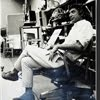## Eugene Ray SDSU 1970'sEugene Ray 1970's. photo credit: Tom Davis

## Friday, May 31, 2013

### POIGNANT MEMOIR=FINAL SDSU EPOCH+A BURST OF LIGHT-1994+
>before all went black<
^^^^^^^^^^^^^^^^
+TONIGHT'S PHOTOS+
>Edward Bourke had been a great student assistant<
>and his younger brother Jim took over helping<
>me w/ the same talent in that last epoch<
^^^^^^^^^^^^^^^^^^^^^^^^^^^^^^^^

#1)                 +MARIAN & I =1994+
>Jim Bourke took this photo of Marian and I in<
>Switzerland at the Rudolf Steiner School<
>where i had gone for a conference<
^^^^^^^^^^^^^^^^^^^^^^^^^^^^^
+GOETHEANUM+
>was a prestigious place to lecture and we<
>also installed a representative group<
^^^^^^^^^^^^^^^^^^^^^^^^

#2)           +SDSU=ED=STUDIO 1994+
>this was the "nerve center" of our last days<
>and the source of our final exhibition<
>for the Bucky Fuller fFoundation<
^^^^^^^^^^^^^^^^^^^^^^^^
( >many exhibition furniture parts<
>i had designed for our large<
+ECCENTRIC PLACES+
>exhibition of the late eighties<)
^^^^^^^^^^^^^^^^^^^^^^

#3)            +TENSILE STRUCTURE+
>won our trophy at San Luis Obispo<
+RESILIENCE IMPORTANT+
>bamboo framed tensile fabric panels with<
>Synergetic lighting in a student design<
>was a typical "hands on" solution<
^^^^^^^^^^^^^^^^^^^^^^^^^

#4)             +DAILY NOTEBOOK+
>someday, after i leave this earth, a complete<
>review of my daily design thinking can be<
>found in the pages of essay books<
^^^^^^^^^^^^^^^^^^^^^^^^^^^^
+ALL STRUCTURES AND+
+LECTURES ARE HERE+
^^^^^^^^^^^^^^^^^

#5)             +LAST EXHIBITIONS+
>still were energized by the inventions that<
>is alive well and growing as technology<
>makes possible all we can imagine<
^^^^^^^^^^^^^^^^^^^^^^^^^^^^
+REMOTE VIEWING+
>and<
+GENETIC MEMORY+
>are proven phenomena and have occupied<
>my thinking and writing for some time<
^^^^^^^^^^^^^^^^^^^^^^^^^^^^
+SHELL STRUCTURES+
>and domes are intelligent concepts for<
+DISASTER RESISTANCE+
^^^^^^^^^^^^^^^^^^^
+1969-1996+
+SDSU=ED PREDICTED THIS+
+SOCIETY IS BACKWARD+
^^^^^^^^^^^^^^^^^^
gene ray, lajolla, california

>eugene ray, mfa, architect<
>professor emeritus, sdsu<